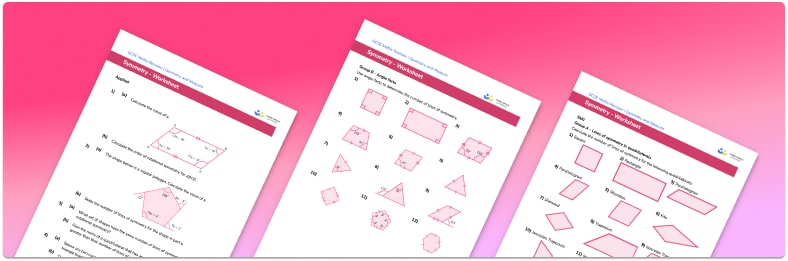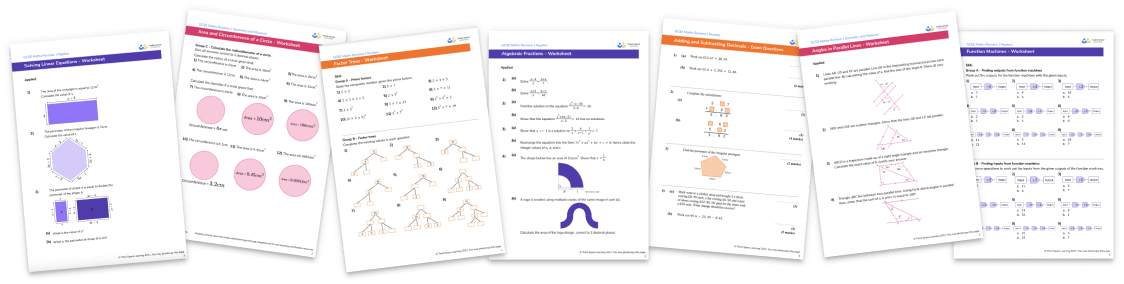# Types Of Angles Worksheet• Section 1 of the types of angles worksheet contains 21 skills-based  types of angles questions, in 3 groups to support differentiation
• Section 2 contains 3 applied types of angles questions with a mix of worded problems and deeper problem solving questions
• Section 3 contains 3 foundation and higher level GCSE exam style questions
• Answer keys and a mark scheme for all questions are provided
• Questions follow variation theory with plenty of opportunities for students to work independently at their own level
• All questions created by fully qualified expert secondary maths teachers
• Suitable for GCSE maths revision for AQA, OCR and Edexcel exam boards

• This field is for validation purposes and should be left unchanged.

You can unsubscribe at any time (each email we send will contain an easy way to unsubscribe). To find out more about how we use your data, see our privacy policy.

### Types of angles at a glance

An angle is formed when two line segments or rays intersect at a vertex. It is important to be able to identify and name angles based on their properties.

We classify angles based on their size. The different types of angle required at GCSE are acute, obtuse, reflex and right angle. An angle measuring less than 90° is an acute angle, greater than 90° but less than 180° is an obtuse angle, and greater than 180° is a reflex angle. An angle of exactly 90° is called a right angle, an angle of exactly 180° is called a straight angle or half turn and an angle of exactly 360° is called a full angle or full turn.

Drawing angles and measuring angles is done using a protractor which can help when identifying angles. It may require rounding decimal answers to the nearest whole degree.

Complementary angles are a pair of angles whose sum is 90°, and supplementary angles are a pair of angles whose sum is 180°. Students are not required to know the terminology of complementary or supplementary angles at GCSE, but they are required to use these facts to calculate missing angles.

Looking forward, students can then progress to additional angles worksheets and other geometry worksheets, for example an angles in polygons worksheet or volume and surface area of spheres worksheet.For more teaching and learning support on Geometry our GCSE maths lessons provide step by step support for all GCSE maths concepts.

## Do you have KS4 students who need more focused attention to succeed at GCSE?There will be students in your class who require individual attention to help them succeed in their maths GCSEs. In a class of 30, it’s not always easy to provide.

Help your students feel confident with exam-style questions and the strategies they’ll need to answer them correctly with our dedicated GCSE maths revision programme.

Lessons are selected to provide support where each student needs it most, and specially-trained GCSE maths tutors adapt the pitch and pace of each lesson. This ensures a personalised revision programme that raises grades and boosts confidence.

Find out more